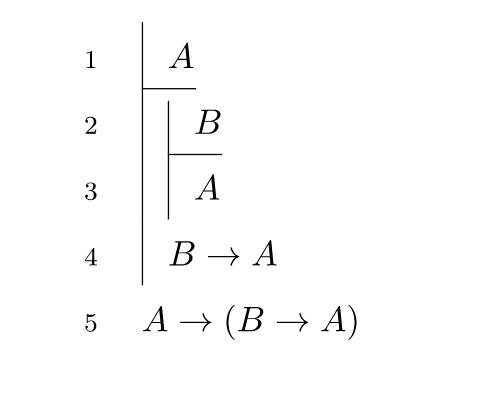# Sprint Preview: April 16–27

Originally published at: https://pressbooks.org/blog/2018/04/16/sprint-preview-april-16-27/

Here’s what we’re working on this sprint: Further Buckram development, approaching 1.0! Implementation of a feature to compare a cloned chapter with its source, as described previously. Integration testing of WP QuickLaTeX. And other bug fixes and improvements as we find them!

Ned,

Thanks for these previews of sprints.

I’m very interested in the QuickLaTeX integration. I’m working on formatting a logic textbook in Pressbooks, and am using WP QuickLaTeX on my server because its the only way I was going to be able to create the Fitch proofs. (See examples below)So one thing that has already been in my head is, how will I communicate to people who clone this book, how to get these proofs to display correctly? Should I create an afterward especially for people that clone my book, with the exact packages and languages that are required to get the proofs to render correctly. With the idea that they can copy and paste into their QuickLaTex advanced settings page? Are you envisioning Pressbooks adopting parts of this plugin, in which case would there be a way to include that kind of information into the cloning process?

As a reference this is the required text on my advanced settings page, it goes beyond just pulling certain packages, because the package I really needed {fitch} isn’t included in the distribution that QuickLaTex’s servers use.

I recognize this is a specialized case, it’s ok if the answer is, we are focusing on more standard use cases.

\usepackage{amsmath}
\usepackage{amsfonts}
\usepackage{amssymb}
\usepackage{mdwtab}
\usepackage{ifthen}
\usepackage{latexsym}

% Line height in proofs
\newlength{\fitchlineht}
\setlength{\fitchlineht}{1.5\baselineskip}
% Horizontal indent between proof levels
\newlength{\fitchindent}
\setlength{\fitchindent}{0.7em}
% Indent to comment
\newlength{\fitchcomind}
\setlength{\fitchcomind}{2em}
% Line number width
\newlength{\fitchnumwd}
\setlength{\fitchnumwd}{1em}

% Altered from mdwtab.sty: shorter vline, for start of subproof
\makeatletter
\newcommand\fvline[\arrayrulewidth]{\vrule@height.5\fitchlineht@width#1\relax}
\makeatother
% Ordinary vertical line
\newcommand{\fa}{\vline\hspace*{\fitchindent}}
% Vertical line, shorter: Use at start of (sub)proof
\newcommand{\fb}{\fvline\hspace*{\fitchindent}}
% Hypothesis
\newcommand{\fh}{\fvline%
\makebox[0pt][l]{{%
\raisebox{-1.4ex}[0pt][0pt]{\rule{1.5em}{\arrayrulewidth}}}}%
\hspace*{\fitchindent}}
% Hypothesis, with longer vert line: for >1 hypothesis
\newcommand{\fj}{\vline%
\makebox[0pt][l]{{%
\raisebox{-1.4ex}[0pt][0pt]{\rule{1.5em}{\arrayrulewidth}}}}%
\hspace*{\fitchindent}}
% Modal subproof: takes argument = operator
\newcommand{\fitchmodal}{%
\makebox[0pt][r]{${}^{#1}$,}\fvline\hspace*{\fitchindent}}
\newcommand{\fn}{\fitchmodal{\Box}}% Box subproof
\newcommand{\fp}{\fitchmodal{\Diamond}}% Diamond subproof
% Modal subproof with hypothesis in first line (as in Fitch)
\newcommand{\fitchmodalh}{%
\makebox[0pt][r]{${}^{#1}$,}%
\fvline%
\makebox[0pt][l]{{%
\raisebox{-1.4ex}[0pt][0pt]{\rule{1.5em}{\arrayrulewidth}}}}%
\hspace*{\fitchindent}}
% Rule: formula introduction marker. \fr with line, \fs without line
\newcommand{\fr}{%
\makebox[0pt][r]{${\rhd}$,,}\vline\hspace*{\fitchindent}}
\newcommand{\fs}{%
\makebox[0pt][r]{${\rhd}$,,}}
% Box around argument, like new variable in ql
\newcommand{\fw}{\fbox{\footnotesize $#1$}}

%
\newcounter{fitchcounter}
\setcounter{fitchcounter}{0}
%To avoid starting from 1, \setboolean{resetfitchcounter}{false}
\newboolean{resetfitchcounter}
\setboolean{resetfitchcounter}{true}
%To avoid increasing numbers, \setboolean{increasefitchcounter}{false}
\newboolean{increasefitchcounter}
\setboolean{increasefitchcounter}{true}
%\formatfitchcounter can be altered if need be, though only once per proof
\newcommand{\formatfitchcounter}{\scriptsize \arabic{#1}}
%Typeset the counter
\newcommand{\fitchcounter}{%
\formatfitchcounter{fitchcounter}}

%A line with a special number – a tag, e.g. \ftag{\vdots}{}
\newcommand{\ftag}{\multicolumn{1}%
{!{\makebox[\fitchnumwd][r]{#1}\hspace{\fitchindent}}Ml@{\hspace{\fitchcomind}}}%
{#2}}

\newenvironment{fitchnum}%
{\ifthenelse{\boolean{resetfitchcounter}}{\setcounter{fitchcounter}{0}}{}
\begin{tabular}{!{\makebox[\fitchnumwd][r]{\fitchcounter }\hspace{\fitchindent}}Ml@{\hspace{\fitchcomind}}l}}%
{\end{tabular}}

\newenvironment{fitchunum}%
{\begin{tabular}{!{\makebox[\fitchnumwd][r]{}\hspace{\fitchindent}}Ml@{\hspace{\fitchcomind}}l}}%
{\end{tabular}}

\newenvironment{fitch}{\renewcommand{\arraystretch}{1.5}
\begin{fitchnum}}{\end{fitchnum}}
\newenvironment{fitch*}{\renewcommand{\arraystretch}{1.5}
\begin{fitchunum}}{\end{fitchunum}}

% The following is useful for giving a numbered formula, then the proof.
\newenvironment{flem}%
{\begin{eqnarray}
\label{#2}\
&\begin{fitch}}%
{\end{fitch}\notag\end{eqnarray}}

%To write comment field for two consecutive lines, with brace
\newcommand{\ftwocom}{%
\parbox[t]{3cm}{
\raisebox{-.6\baselineskip}[\baselineskip][0pt]{%
\left. \begin{aligned} ,\ , \end{aligned} \right}\quad #1}
}}